StatLect

# Alternative hypothesis

In a statistical test, observed data is used to decide whether or not to reject a restriction on the data-generating probability distribution.

The assumption that the restriction is true is called null hypothesis, while the statement that the restriction is not true is called alternative hypothesis.

A correct specification of the alternative hypothesis is essential to decide between one-tailed and two-tailed tests.## Notation

Usually, the null hypothesis is denoted by, while the alternative hypothesis is denoted by.

## Mathematical setting

In order to fully understand the concept of alternative hypothesis, we need to remember the essential elements of a statistical inference problem:

• we observe a sample drawn from an unknown probability distribution;

• in principle, any valid probability distribution could have generated the sample;

• however, we usually place some a priori restrictions on the set of possible data-generating distributions;

• the restricted set of distributions, denoted by, is called a statistical model.

A couple of simple examples follow.

Example If we assume that the data are drawn from a normal distribution, then the statistical modelis the set of all normal distributions.

Example Suppose that the data are extracted from a distribution with unit variance. Thenis the set of all probability distributions that have unit variance.

## Definition

When we conduct a statistical test, we formulate a null hypothesis as a restriction on the statistical model.

Denote bythe true data-generating distribution.

The null hypothesis can be expressed as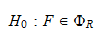whereis a subset of.

The alternative hypothesis is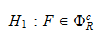where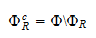is the complement of, that is, the subset ofthat comprises all the distributions not belonging to.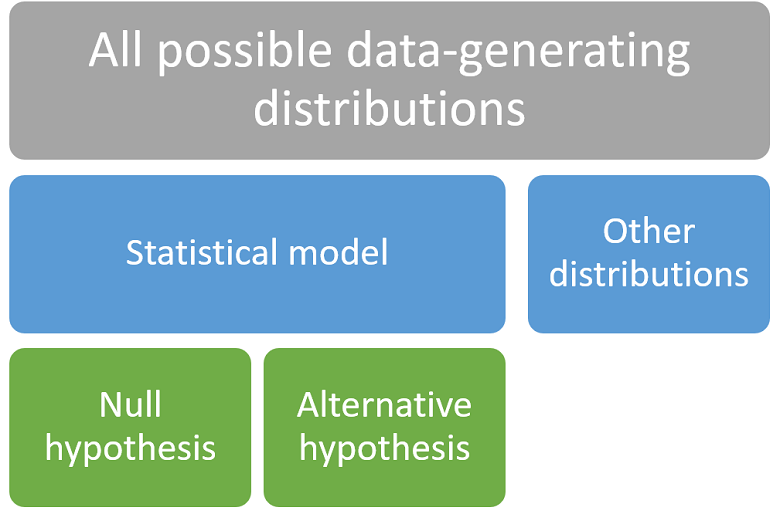## Example

Let the statistical modelbe the set of all normal distributions.

The null hypothesis is that the meanof the data-generating distribution is positive.

Thenis the set of all normal distributions with positive mean.

For simplicity, we write the null hypothesis asIt follows thatis the set of all normal distributions with strictly negative mean.

We write the alternative hypothesis as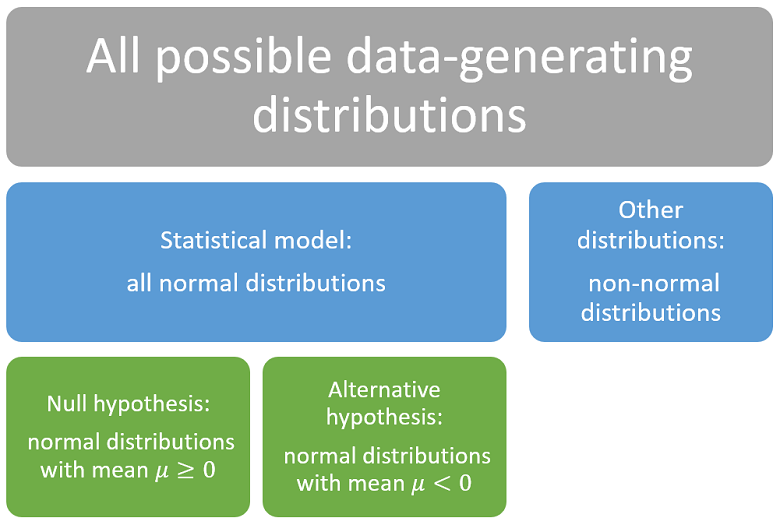## Choice between one-tailed and two-tailed tests

The alternative hypothesis is used to decide whether a test should be one-tailed or two-tailed.

### The critical region

The null hypothesis is rejected if the test statistic falls within a critical region that has been chosen by the statistician.

The critical region is a set of values that may comprise:

• only the left tail of the distribution or only the right tail (one-tailed test);

• both the left and the right tail (two-tailed test).

The choice of the critical region depends on the alternative hypothesis. Let us see why.

### The interpretation of the rejection

Usually, when the test statistic falls within the critical region, the rejection of the null hypothesis is clearly interpreted as evidence that the data-generating distributioncomes from a set of distributionsthat is disjoint from(remember that). The subscriptstands for interpretation.

The interpretation is different depending on the tail of the distribution in which the test statistic falls.

Example Consider a test of hypothesis for the mean of a normal distribution, where we test. The test statistic is the z-statistic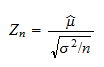whereis the sample mean,is the variance of the distribution andis the sample size. If we run a two-tailed test with critical value, the critical regionis the union of the right and left tails of the distribution of: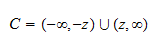The test statistic falls in the right tail (i.e.,) when the sample meanis much larger than zero. In this case, the rejection of the null hypothesis is interpreted as evidence that, most likely,; the set of distributionsis the set of all normal distributions with strictly positive mean.

### The interpretation must be coherent with the alternative hypothesis

The choice between a one-tailed or a two-tailed test needs to be done in such a way that the interpretation of a rejection is always coherent with the alternative hypothesis.

In other words, we must ensure thatExample As in the previous example, consider a test about the mean of a normal distribution, where we test. Suppose that we exclude a priori thatcan be negative. In other words, the statistical modelincludes all the normal distributions with mean. It follows thatincludes all the normal distributions withand the alternative hypothesis is. Can we use a two-tailed z-test? No because if the sample mean is much lower than zero and the test statistic falls within the left tail, then the interpretation is that, most likely,. But this was excluded a priori in the formulation of the statistical model. Thus, the interpretation would be incoherent with the alternative hypothesis:would not be included in. As a consequence, we need to perform a one-tailed test, in which the critical region includes only the right tail.

## Power function

When we deal with the power function of a test, the term "alternative hypothesis" has a special meaning.

Suppose that we are conducting a test about a parameter, in which the null hypothesis is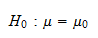The power functionis a function that gives, for any, the probability of rejectingwhen the true parameter is equal to.

The argumentis often called alternative hypothesis andis called power against the alternative.

## Accepting the alternative

We conclude with a caveat about the interpretation of the outcome of a test of hypothesis.

The interpretation of a rejection of the null is controversial.

According to some statisticians, rejecting the null is equivalent to accepting the alternative.

However, others deem that rejecting the null does not necessarily imply accepting the alternative. In fact, it is possible to think of situations in which both hypotheses can be rejected. Let us see why.

According to the conceptual framework illustrated by the images above, there are three possibilities:

1. the null is true;

2. the alternative is true;

3. neither the null nor the alternative is true because the true data-generating distribution has been excluded from the statistical model (we say that the model is mis-specified).

If we are in case 3, accepting the alternative after a rejection of the null is an incorrect decision. Moreover, a second test in which the alternative becomes the new null may lead us to another rejection.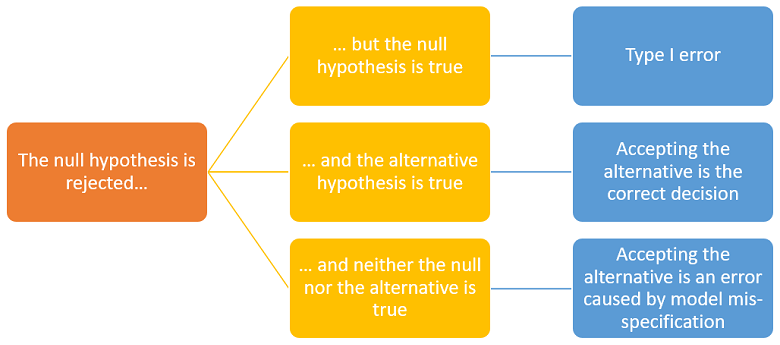## More details

You can find more details about the alternative hypothesis in the lecture on Hypothesis testing.

Previous entry: Almost sure

Next entry: Binomial coefficient